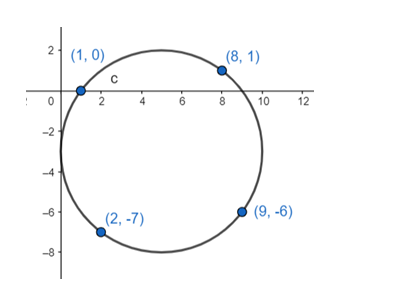# Show that the points`
Question:

Show that the points $A(1,0), B(2,-7), c(8,1)$ and $D(9,-6)$ all lie on the same circle. Find the equation of this circle, its centre and radius.

Solution:The general equation of a circle: $(x-h)^{2}+(y-k)^{2}=r^{2}$

...(i), where $(\mathrm{h}, \mathrm{k})$ is the centre and $\mathrm{r}$ is the radius.

Putting $(1,0)$ in (i)

$(1-h)^{2}+(0-k)^{2}=r^{2}$

$\Rightarrow \mathrm{h}^{2}+\mathrm{k}^{2}+1-2 \mathrm{~h}=\mathrm{r}^{2} . .$ (ii)

Putting $(2,-7)$ in (i)

$(2-h)^{2}+(-7-k)^{2}=r^{2}$

$\Rightarrow h^{2}+k^{2}+53-4 h+14 k=r^{2}$

$\Rightarrow\left(h^{2}+k^{2}+1-2 h\right)+52-2 h+14 k=r^{2}$

$\mathrm{h}-7 \mathrm{k}-26=0$..(iii) [from (ii)]

Similarly putting (8, 1)

$7 \mathrm{~h}+\mathrm{k}-32=0$..(iv)

Solving (iii)&(iv)

h = 5 and k = - 3

centre(5, - 3)

To check if $(9,-6)$ lies on the circle, $(9-5)^{2}+(-6+3)^{2}=5^{2}$